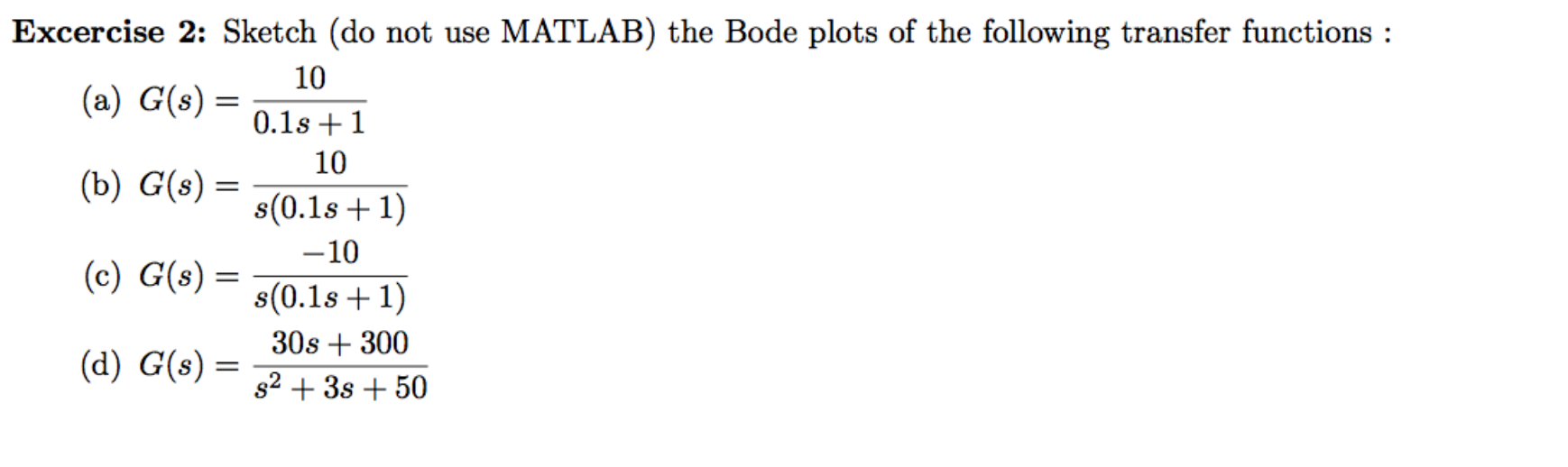# Excercise 2: Sketch (do not use MATLAB) the Bode plots of the following transfer functions:10(a) G(s)0.1s110(b) G(s) =s(0.1s 1)-10(c) G(s)s(0.1s 1)30s300(d) G(s)s23s50

Questionhelp_outlineImage TranscriptioncloseExcercise 2: Sketch (do not use MATLAB) the Bode plots of the following transfer functions: 10 (a) G(s) 0.1s1 10 (b) G(s) = s(0.1s 1) -10 (c) G(s) s(0.1s 1) 30s300 (d) G(s) s23s50 fullscreen
check_circle

Step 1

Since we only answer up to 3 sub-parts, we’ll answer the first 3. Please resubmit the question and specify the other subparts (up to 3) you’d like answered.

Step 2

(a)

Time constant form of transfer function is –

Step 3

Value of K from transfer function is 10.

One pole is at S=10.

In bode plot, for each pole there is a change of slope of -20dB/decade ...

### Want to see the full answer?

See Solution

#### Want to see this answer and more?

Solutions are written by subject experts who are available 24/7. Questions are typically answered within 1 hour.*

See Solution
*Response times may vary by subject and question.
Tagged in

### Electrical Engineering